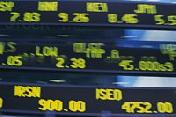Financial Terms Certainty equivalent

Definition of Certainty equivalentCertainty equivalent

An amount that would be accepted in lieu of a chance at a possible higher, but
uncertain, amount.

Related Terms:

A probability used to determine a "sure" expected value (sometimes called a
certainty equivalent) that would be equivalent to the actual risky expected value.

Bond-equivalent basis

The method used for computing the bond-equivalent yield.

Bond equivalent yield

Bond yield calculated on an annual percentage rate method. Differs from annual
effective yield.

Bond-equivalent yield

The annualized yield to maturity computed by doubling the semiannual yield.

Bond Equivalent Yield

Bond yield calculated on an annual percentage rate method

CASH AND CASH EQUIVALENTS

The balance in a company’s checking account(s) plus short-term or temporary investments (sometimes called “marketable securities”), which are highly liquid.

Cash and equivalents

The value of assets that can be converted into cash immediately, as reported by a
company. Usually includes bank accounts and marketable securities, such as government bonds and Banker's
Acceptances. Cash equivalents on balance sheets include securities (e.g., notes) that mature within 90 days.Cash equivalent

A short-term security that is sufficiently liquid that it may be considered the financial
equivalent of cash.

Cash-equivalent items

Temporary investments of currently excess cash in short-term, high-quality
investment media such as treasury bills and Banker's Acceptances.

Cash Equivalents

Highly liquid, fixed-income investments with original maturities of three months or less.

Cash Equivalents

Instruments or investments of such high liquidity and safety that they are virtually equal to cash.

Common stock equivalent

A convertible security that is traded like an equity issue because the optioned

Corporate taxable equivalent

Rate of return required on a par bond to produce the same after-tax yield to
maturity that the premium or discount bond quoted would.

Coupon equivalent yield

True interest cost expressed on the basis of a 365-day year.

Equivalent annual annuity

The equivalent amount per year for some number of years that has a present
value equal to a given amount.

Equivalent annual benefit

The equivalent annual annuity for the net present value of an investment project.

Equivalent annual cash flow

Annuity with the same net present value as the company's proposed investment.

Equivalent annual cost

The equivalent cost per year of owning an asset over its entire life.

equivalent annual cost

The cost per period with the same present value as the cost of buying and operating a machine.

Equivalent bond yield

Annual yield on a short-term, non-interest bearing security calculated so as to be
comparable to yields quoted on coupon securities.

Equivalent loan

Given the after-tax stream associated with a lease, the maximum amount of conventional
debt that the same period-by-period after-tax debt service stream is capable of supporting.

Equivalent taxable yield

The yield that must be offered on a taxable bond issue to give the same after-tax
yield as a tax-exempt issue.

equivalent units of production (EUP)

an approximation of the number of whole units of output that could have been
produced during a period from the actual effort expended
during that period; used in process costing systems to assign
costs to production

Fixed-income equivalent

Also called a busted convertible, a convertible security that is trading like a straight
security because the optioned common stock is trading low.

Inflation uncertainty

The fact that future inflation rates are not known. It is a possible contributing factor to
the makeup of the term structure of interest rates.